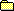# JavaScript语言参考手册_核心

#### JavaScript

 JavaScript手册目录此参考中包含的内容轻松上手简介操作符语句核心文档窗口表单浏览器事件和事件句柄LiveWire数据库服务进程管理服务实用工具全局函数LiveConnect的Java包索引版权
【目录】 【上一页】 【下一页】 【索引】

# Number

Lets you work with numeric values. The Number object is an object wrapper for primitive numeric values.

#### 创建源

The Number constructor:

#### 描述

The primary uses for the Number object are:

• To access its constant properties, which represent the largest and smallest represen表 numbers, positive and negative infinity, and the Not-a-Number value.
• To create numeric objects that you can add properties to. Most likely, you will rarely need to create a Number object.
The properties of Number are properties of the class itself, not of individual Number objects.

## MAX_VALUE

The maximum numeric value represen表 in JavaScript.

#### 描述

The MAX_VALUE property has a value of approximately 1.79E+308. Values larger than MAX_VALUE are represented as "Infinity".

#### 示例

The following code multiplies two numeric values. If the result is less than or equal to MAX_VALUE, the func1 function is called; otherwise, the func2 function is called.

## MIN_VALUE

The smallest positive numeric value represen表 in JavaScript.

#### 描述

The MIN_VALUE property is the number closest to 0, not the most negative number, that JavaScript can represent.

#### 示例

The following code divides two numeric values. If the result is greater than or equal to MIN_VALUE, the func1 function is called; otherwise, the func2 function is called.

## NaN

A special value representing Not-A-Number. This value is represented as the unquoted literal NaN.

#### 描述

JavaScript prints the value Number.NaN as NaN.

#### 示例

In the following example, if month has a value greater than 12, it is assigned NaN, and a message is displayed indicating valid values.

#### 参看

isNaN, parseFloat, parseInt

## NEGATIVE_INFINITY

A special numeric value representing negative infinity. This value is displayed as "-Infinity".

#### 描述

This value behaves mathematically like infinity; for example, anything multiplied by infinity is infinity, and anything divided by infinity is 0.

#### 示例

In the following example, the variable smallNumber is assigned a value that is smaller than the minimum value. When the if statement executes, smallNumber has the value "-Infinity", so the func1 function is called.

## POSITIVE_INFINITY

A special numeric value representing infinity. This value is displayed as "Infinity".

#### 描述

This value behaves mathematically like infinity; for example, anything multiplied by infinity is infinity, and anything divided by infinity is 0.

#### 示例

In the following example, the variable bigNumber is assigned a value that is larger than the maximum value. When the if statement executes, bigNumber has the value "Infinity", so the func1 function is called.

## prototype

Represents the prototype for this class. You can use the prototype to add properties or methods to all instances of a class. For information on prototypes, see Function.prototype.

## toString

Returns a string representing the specified object.

toString()
toString(radix)

#### 描述

Every object has a toString method that is automatically called when it is to be represented as a text value or when an object is referred to in a string concatenation.

【目录】 【上一页】 【下一页】 【索引】